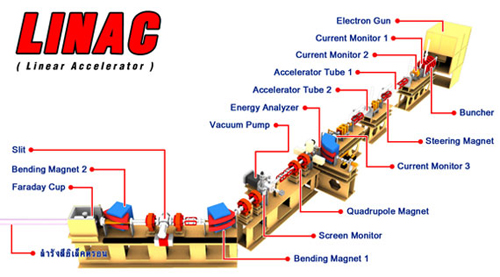Electron gun

• Cathode Model: EIMAC Y646B
• Cathode Heater Current = 1.2-1.4 A.
• Cathode Operating Temperature = 1100 C
• Accelerating High Voltage = 100 kV (Voltage between anode and cathode)
• Grid Transparency = 77 %
• Grid Pulse Voltage = 98 V
• Grid Bias Voltage = -28 V
• Pulse Length = 3
• Current Output from electron gun 100-200 mA
• Repetition Rate = 0.5 Hz

Klystron

• Voltage between Anode and cathode = 270 kV (klystron HV)
• Microwave Power Output = 20 MW
• RF frequency = 2856 MHz

Pre-bunchers

• Pre-buncher #1 : Microwave Power Input = 1.4 kW
• Pre-buncher #2 : Microwave Power Input = 1.4 kW

Buncher

• Microwave power = 3.4 MW
• 9 cavities
• Accelerator type : Standing wave
• Electron Energy Output = 8 MeV

Linear Accelerator Structure #1

• Accelerator tube length = 2.3 m
• 60 cavities of copper disks
• Accelerator type : constant impedance traveling wave LINAC
• Electron Energy Output = 24 MeV